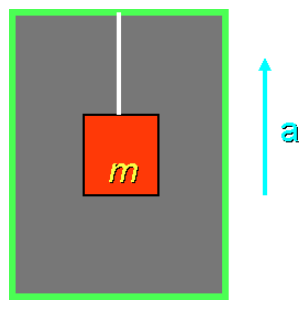# Problem: A box of mass m hangs by a string from the ceiling of an elevator that is accelerating upward.1) Which of the following best describes the tension T in the string?A. T &lt; mgB. T = mgC. T &gt; mg

###### FREE Expert Solution

Newton's second law:

$\overline{){\mathbf{\Sigma }}{\mathbf{F}}{\mathbf{=}}{\mathbf{m}}{\mathbf{a}}}$

The tensional force acts upwards of the box.

The force due to gravity acts downwards of the box.

83% (239 ratings)###### Problem Details

A box of mass m hangs by a string from the ceiling of an elevator that is accelerating upward.1) Which of the following best describes the tension T in the string?

A. T < mg
B. T = mg
C. T > mg